# C++ Object | How to pass and return object from a function in C++ - Learn C++ - C++ Tutorial - C++ programming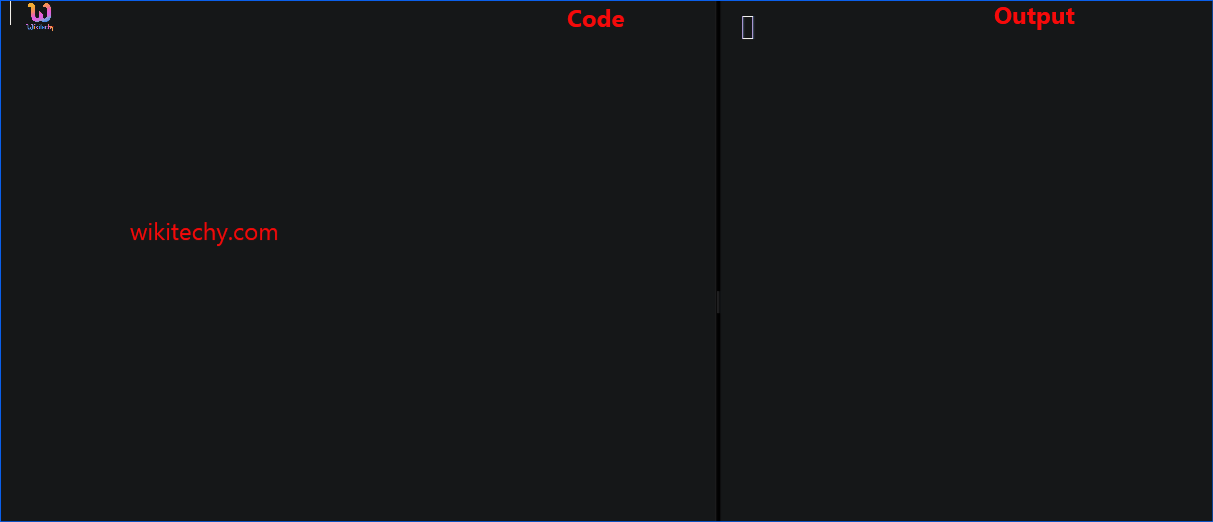Learn c++ - c++ tutorial - c++-object - c++ examples - c++ programs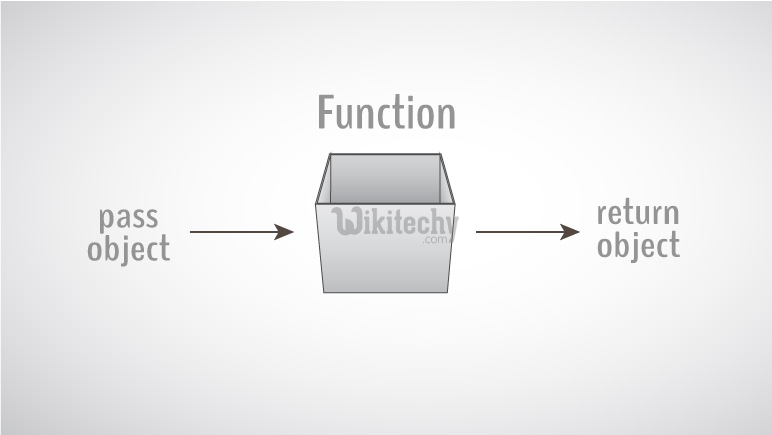• You need to create a new constructor to perform this task. But, no additional constructor is needed.
•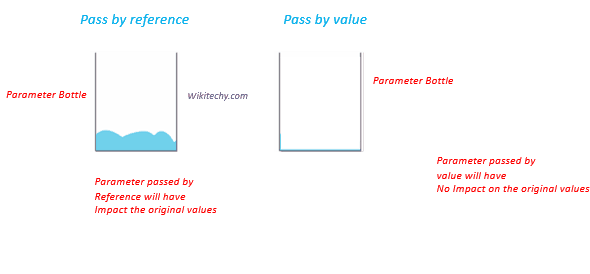learn c++ tutorials - pass by value vs pass by reference in c++ Example

• This is because the copy constructor is already built into all classes by default.
• In C++ programming, objects can be passed to a function in a similar way as structures.

## How to pass objects to a function?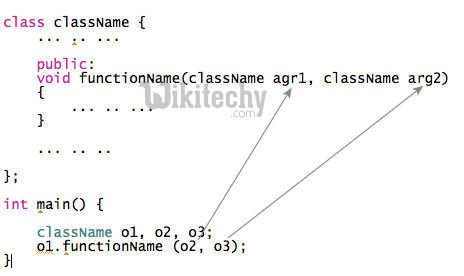## Example 1: Pass Objects to Function

• C++ program to add two complex numbers by passing objects to a function.

## How to return an object from the function?

• In C++ programming, object can be returned from a function in a similar way as structures.
• As the return type of function is weight (an object of class weight), a temporary object temp is created within the function for holding return values. These values are accessed as temp kilogram and temp gram by the function. When the control returns to main (), the object temp is assigned to the object w3 in main( ).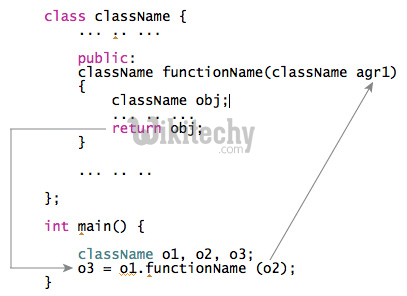## Example 2: Pass and Return Object from the Function

• In this program, the sum of complex numbers (object) is returned to the main() function and displayed.